### File information

This PDF 1.4 document has been generated by TeX / pdfTeX-1.11a, and has been sent on pdf-archive.com on 19/06/2015 at 18:33, from IP address 79.116.x.x. The current document download page has been viewed 491 times.
File size: 43 KB (4 pages).
Privacy: public file

### Document preview

The Fail of Relativity
Octavian Balaci
Calea Caransebesului 11 F 9 Resita Romania
December 5, 2008

Abstract
Reasert the old twin paradox in a new light leading to conclusion
that theory of relativity is inconsistent with physical reality. Also possible alternatives to undestand electrodynamic fenomenons are analysed.

1

The clock paradox also known as twin paradox is a direct consequence of
relativity principle symmetry in the context of constant light speed principle,
with other words in context of special relativity. One consequence of special
relativity is time dilation witch say that in a moving inertial reference frame
with respect to a stationary reference frame, the time intervals become larger
and consequently the clocks run slower than clocks from stationary reference
frame. Such an a¢ rmation that moving clocks runs slover than stationary
clocks, in context of relativity principle is inconsistent because if we consider
relativity principle as a basic principle of physics, then we cannot say that A
is moving and B is sit, but correctly is to say that exist a relativ movement
between A and B or between A and B we have a relative velocity v, as
result while relativity principle is active we cannot have a sense of which
is in motion and have its local time dilated, instead both A and B can be
considered in motion relative to each other, so both have they local time
dilated relativ to each other what is of course impossible.

1.1

Is the best known case of clock paradox, often denominated as twin paradox,
the original two clocks variant is pretty useless because imply accelerations
and fall outside the scope of special relativity, which leave room for various
interpretations from both sides supporters and opponents of relativity. Lets
to suppose we have two clocks A and B, initialy both clocks are in the same
reference frame having the same state of motion. The clocks counters are
1

cleared to 0 and clock B is accelerated at speed v with respect to clock A
which remain in the same state of motion. After a while the clock B stop
and turn back with same speed v with respect to clock A, until it reach
the clock A and the clocks counters are compared. Analyzing the problem
from the clock A reference frame, which is a valid inertial reference frame on
entire duration of experiment, will result that clock B has lag behind clock
A due to kinetic time dilation caused by moving of B with respect to A.
However the same analysis can be made from the clock B reference frame
which see that clock A is moving with respect with B and consequently the
clock A will lag behind clock B due to time dilation. However the problem
is that clock B reference frame experience accelerations and consequently is
not a valid reference frame from special relativity point of view, as result this
case cannot be considered a clear paradox of special relativity. Supporters
of relativity still try to resolve this paradox in a way that uses a light pulses
counting technique, which is noting more than doppler analysis, but time
dilation is a purely kinetic transformational e¤ect and have noting to do
with doppler e¤ect, so this solution is wrong. Others try to take dynamic
e¤ects of acceleration into consideration and move the problem into scope of
general relativity, this way is wrong from start because kinetic time dilation,
as it name imply, have nothing to do with acceleration and gravity. This
classic clock paradox is an asymmetric case which not reveal the inherent
problem of special relativity.

1.2

The simplest symmetric version of clock paradox experiment have only two
clocks and a system to compare the clocks counters without the necessity
to bring the clocks together. Lets suppose we have two very long rods,
every rod have a clock at one end and a marker at other end. The marker
is nothing more than a head (e.g. a small magnet) which can be sensing
by an appropriate sensor embedded in clock, when the clock pass near it.
Now these two rods are already in motion with respect to each other with
speed v on an approaching trajectory with the clocks in front of movement
direction. We arbitrary name them rod system A and rod system B, however
the analysis is perfectly symmetrical.

Figure 1: symmetric 2 clocks experiment

When the clocks reach each other their counters are cleared to 0, from
2

now on we analyze the problem from clock A reference frame, but is the
same from clock B reference frame. When clock B reach the marker a it
send a light pulse (electromagnetic pulse) in direction of clock A followed
by transmision of counter value as was at the moment of marker detection.
When clock A receive the light pulse, it will memorize the value of own
counter at that moment, the clocks counters never stop, and then receive
and memorize the transmitted value of clock B counter. Because the lenght
of the rod is known as L and the clock A and marker a reside in the same
reference frame, using the principle of constant light speed we can calculate
L
the ‡ight time of light pulse as =
and substract it from memorized
c
counter value of clock A to …nd which was the clock A counter value when
clock B counter value was extracted and transmitted. When these two
counters values are compared, the clock A should see, according to relativity,
that clock B lag behind clock A. If the analysis is repeated identical from
clock B reference frame, will result that clock A lag behind clock B, which
is impossible and reveal the paradox. The entire theory of relativity su¤er
from symmetric inconsistencies, what mean that the relativity principle and
constant light speed principle cannot stand together as valid principles of
physics, one or both of them are erroneous. Of course the paradox disapear
if we say that one clock only see the other clock how lag behind due to time
necessary to information carrier (e.g. ligh or sound) to cover the distance
between them, but this is in fact a measurement problem not a physical law.
Seem that Einstein raised at rank of fundamental space-time properties a
simple measurement characteristic using electromagnetic …eld as information
carrier.

1.3

General relativity can save situation?

No, obviously not, why? First because is based on space-time kinetic properties de…ned by special relativity, which is broken, second because the principle of equivalence between inertial and havy mass is no more than a simple
method, a tool, not a physical law. Saying that an accelerated reference
frame will generate a gravitational …eld extended on entire space is obviosly
only a mathematical method, cannot result from a principle of nature because such a …eld will have in…nite energy which is imposible, as result the
so called principle of equivalence of general relativity is in fact a method of
equivalence. Also seem that some astronomic observations indicate a very
high propagation velocity for gravitational …eld, considerably higher than
the propagation velocity for electromagnetic …eld, which contradict the theory of relativity, …rst as a space-time theory and second as a theory of gravity,
eliminating the light speed value c as a limit speed for all interactions. Consequently the general relativity is broken too as fundamental theory, again
Einstein raised at rank of principle of nature a simple methematical method.

3

2

If the relativity is eliminated then some modern scienti…c theories are also
eliminated and the theoretical physics need to reanalyse many concepts from
begining of twenty century. Also the relativity theory was intended to be
the missing link of electrodynamic, which reconcile the …eld view of electromagnetic fenomenons with kinetic concept of mechanics, especialy with
relativity of movement.

References
 Albert Einstein (1905) "On the Electrodynamics of Moving Bodies"
 Albert Einstein (1922) "Sidelights on Relativity"
 William H. McCrea (1951) "The Clock Paradox in Relativity Theory"
 Herbert Dingle (1956) "A Problem in Relativity Theory"
 Herbert Dingle (1972) "Science at the Crossroads"
 Luis Essen (1971) "The Special Theory of Relativity: A Critical Analysis"
 Tom Van Flandern (1998) "The Speed of Gravity – What the Experiments Say"
 Milan R. Pavlovic (2000) "Einstein’s Theory of Relativity - Scienti…c
Theory or Illusion?"
 Ling Jun Wang (1999) "Symmetrical Experiments to Test the Clock

4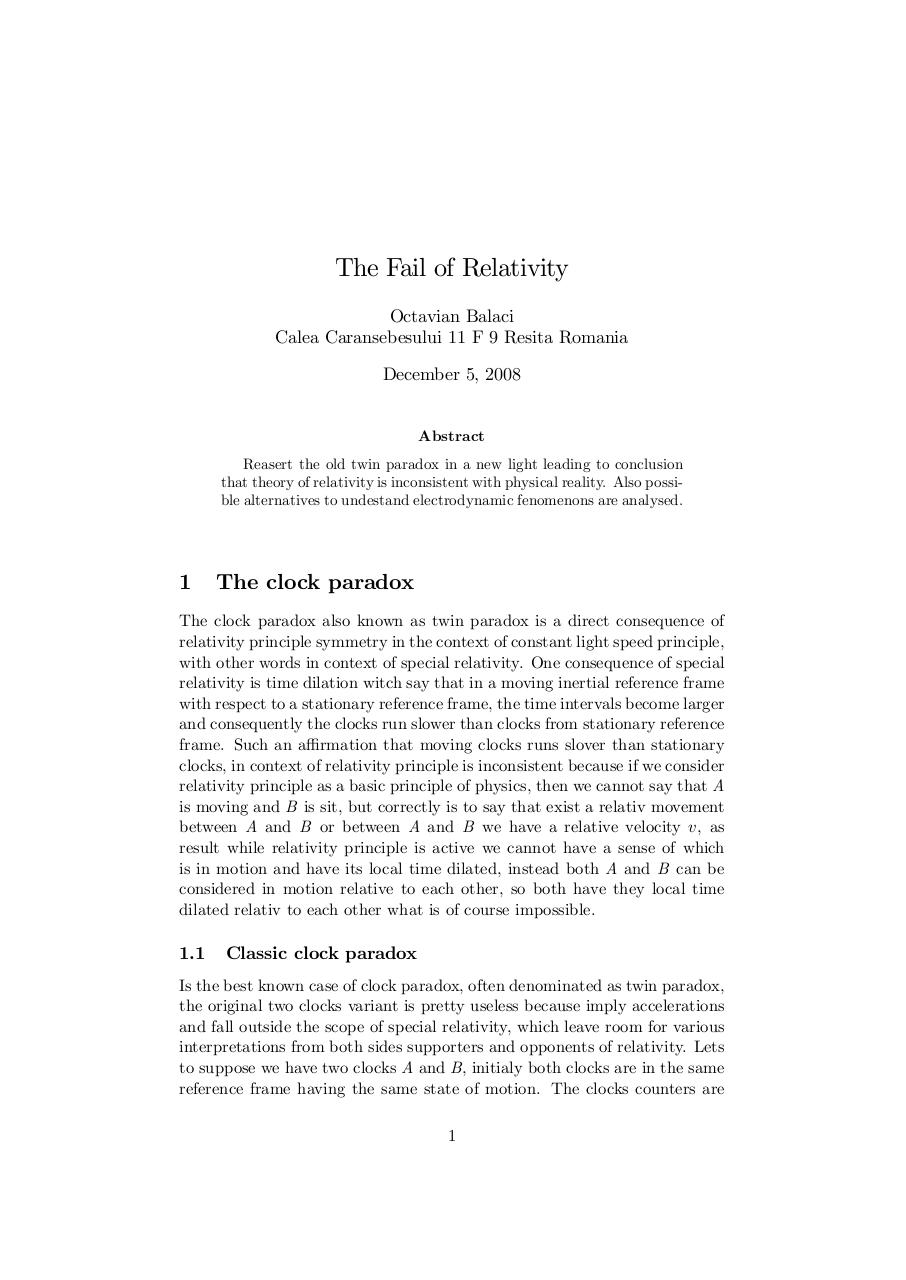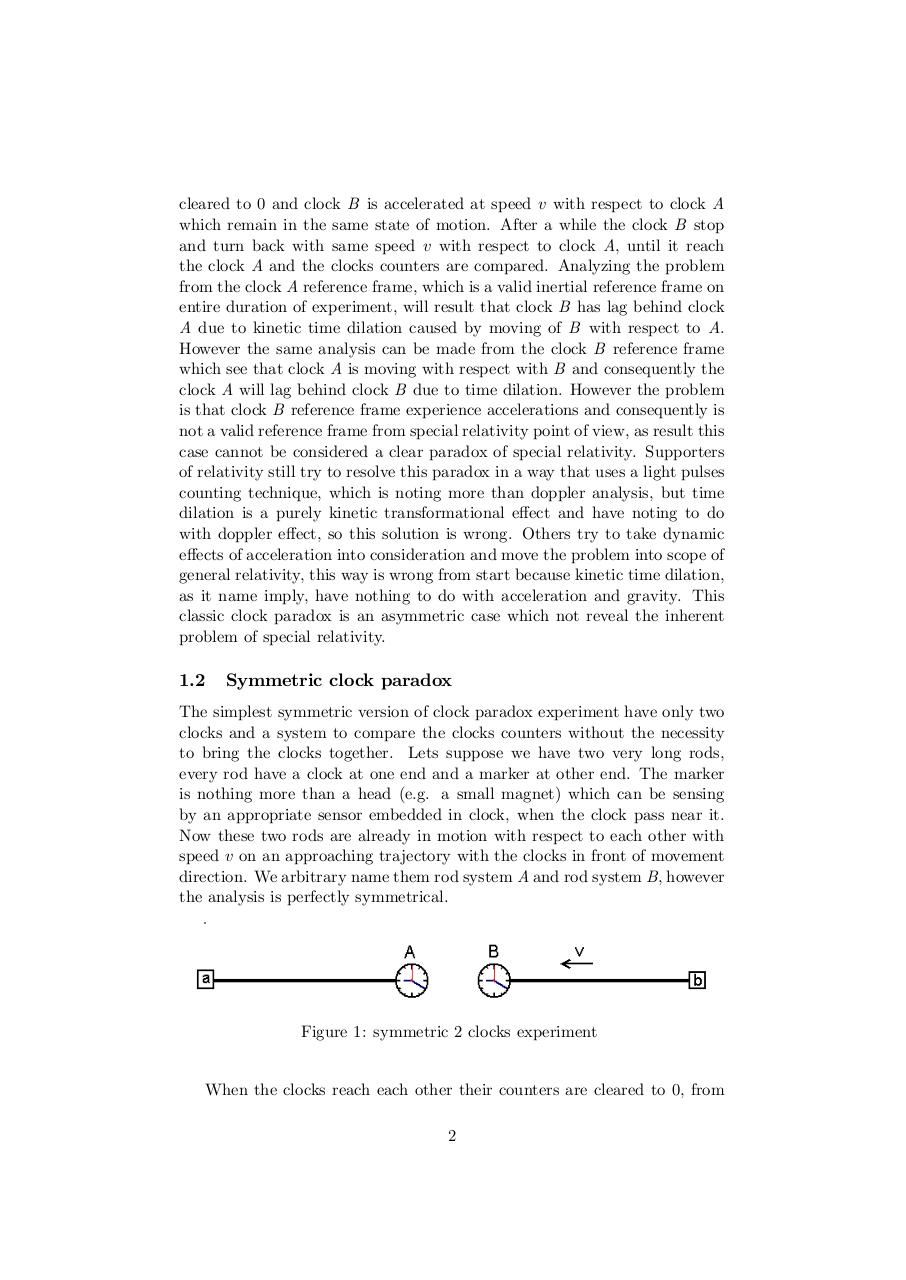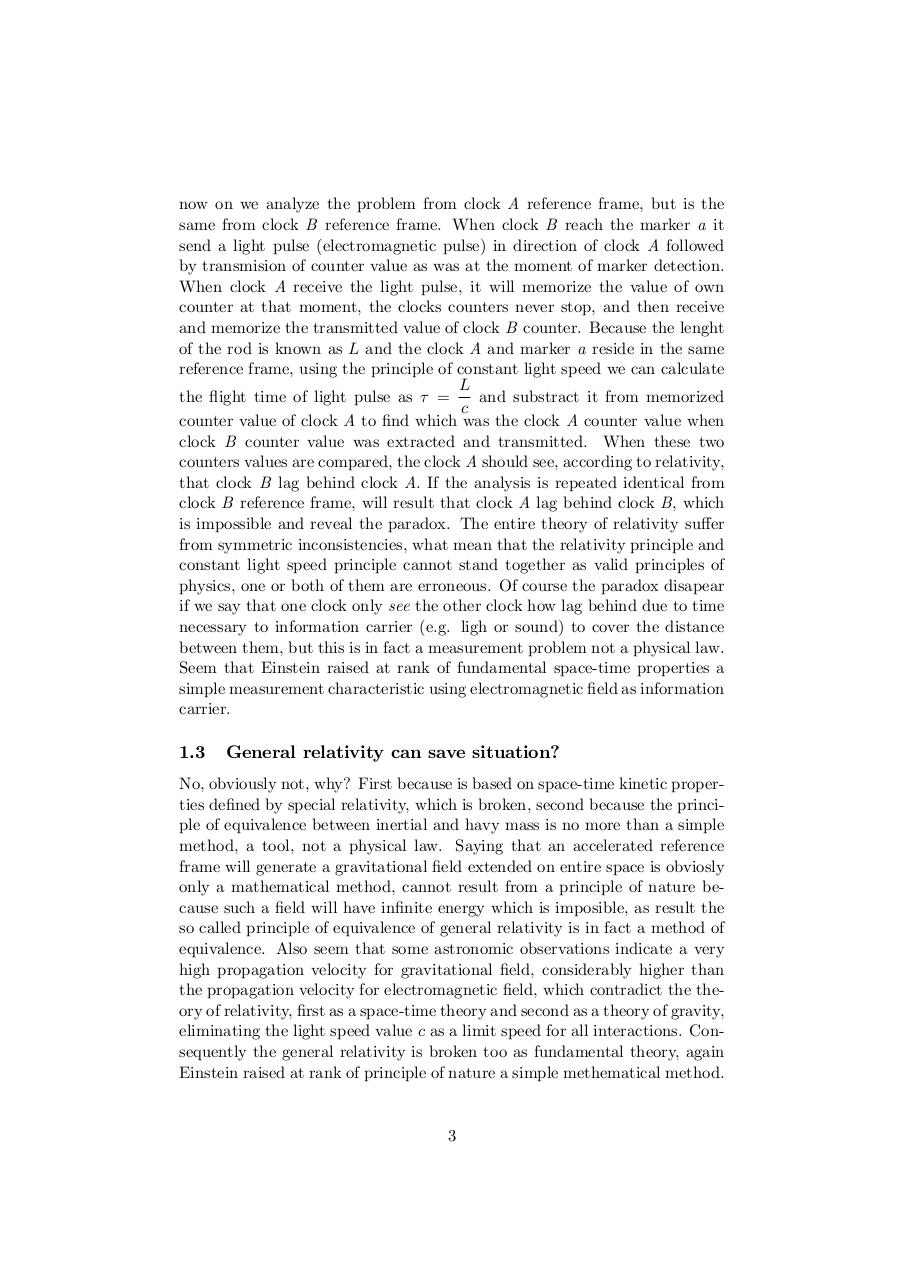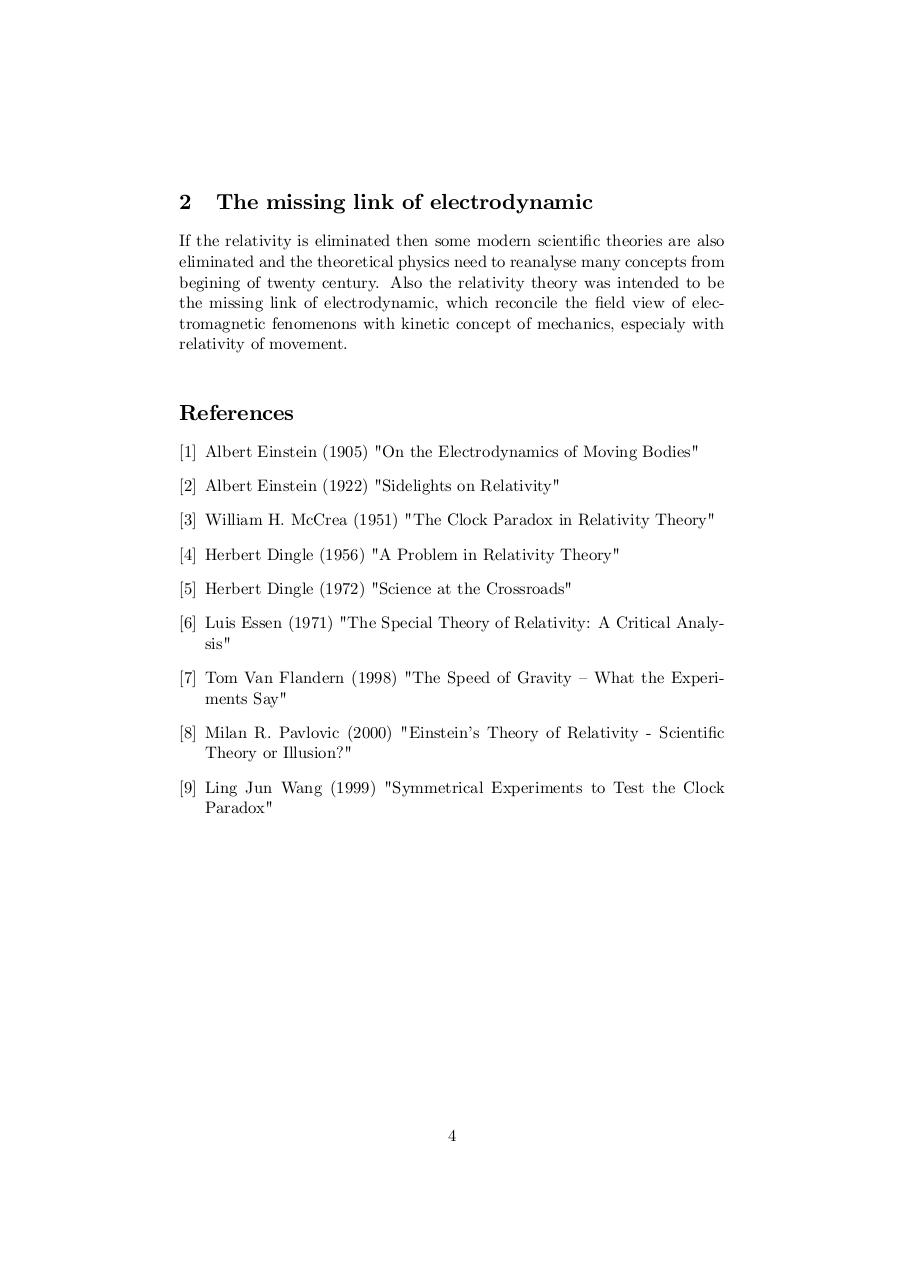#### HTML Code

Copy the following HTML code to share your document on a Website or Blog

#### QR Code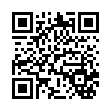### Related keywords